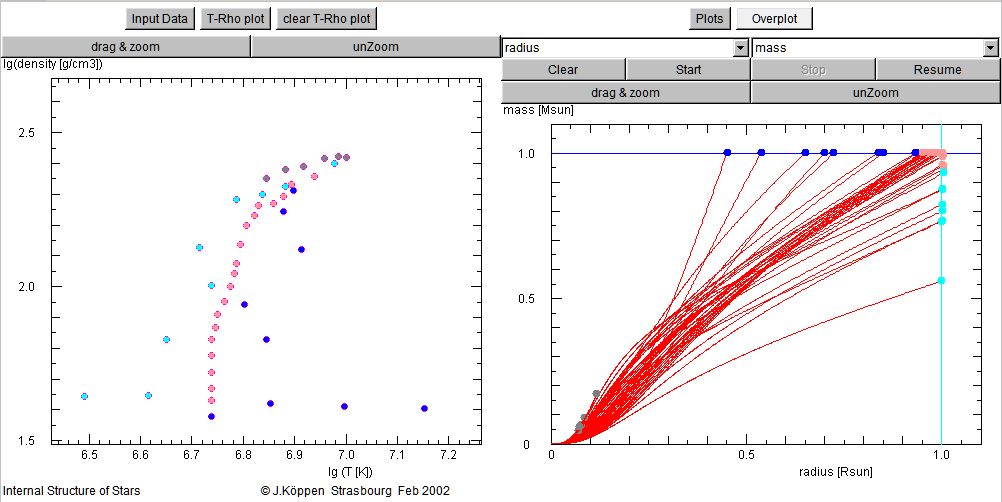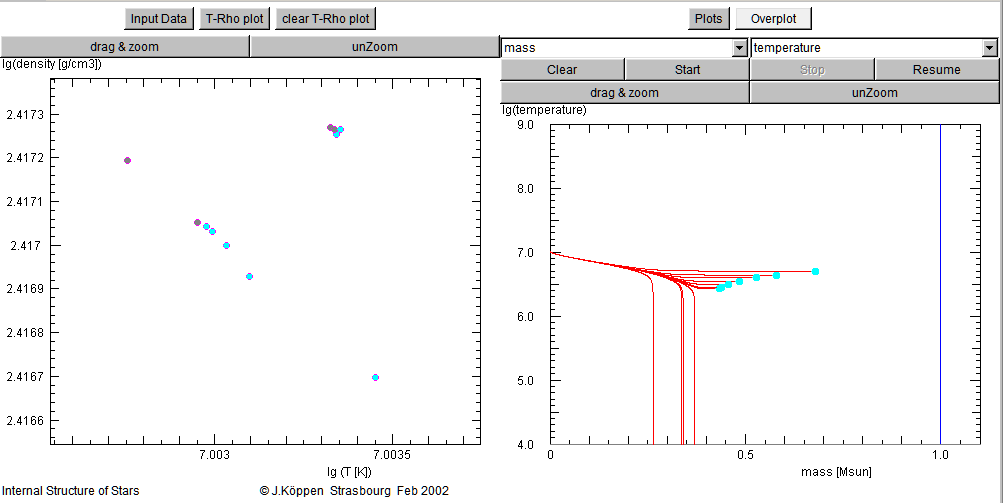Internal Structure of Stars

Joachim Köppen Kiel/Strasbourg March 2013

Warning: some of the features of this applet are not fully functional yet! Also, the opacity table is rather simple, so that radiative and convective equilibrium models are only very approximate!!

Some quick explanations of the applet's use:

Left hand buttons

• Input Data: shows the input parameter page
• T-Rho plot: shows the plot of central temperature and density, which is used to start the simulation
• clear T-Rho plot
T-Rho Plot
• to operate, select on the right hand side e.g. the radius-mass plot; clicking on the T-Rho plot will start the computation, whose result is displayed on the radius-mass plot. The aim is to find the initial T-Rho combination which ends up in the desired mass at the desired stellar radius. The initial dot is marked with a colour depending on the outcome.
• blue = stellar mass reached
• cyan = stellar radius reached
• green = low surface pressure reached
• gray = low surface temperature reached
• shades of red indicate that several surface conditions have simultaneously been reached
• the parameter values of the last model are displayed in TextFields on the input parameter page, where they can be modified manually ...
Left hand Page
• Model Type: here one can choose between isothermal, polytropic, purely radiative, and radiative/convective models
• hitting enter key in the following TextFields starts computation
• comput.accuracy: a tolerance for the radial step width
• stellar mass
• polytropic index
• opacity: this scaling factor has been set to give a solar model in radiative equilibrium ...
• central temperature
• helium mass fraction
• metal mass fraction

• last x guess: the parameter (T or K) of last simulation
• last y guess: log(central_density) of last simulation

• stellar radius: shows the result of last simulation
• stellar luminos.: shows the result of last simulation
Right hand buttons
• Overplot: switches on/off the overplotting of all curves
• clear: erases all present curves and dots
• start: (re-)starts the simulation with the last guess parameters given on the left hand panel (or from the T-Rho plot)
• stop: halts the current simulation
• resume: continues with the halting simulation
• drag&zoom: click the button, then drag the mouse over that region of the plot which you want to zoom in. Then re-run the simulation
• unZoom: brings back the full view of the plot, but you have to re-run to restore the curve(s)
Right hand plots
• Plots: does not do anything (yet)
• Sing.Curve: switches on/off the overplotting of all curves
• the abscissa and ordinate can be chosen among these variables: radius, mass, density, pressure, temperature, luminosity, energy fraction from CNO cycle, ratio of radiation pressure and gas pressure, fraction of convective flux (note: some of the features are not yet implemented).For an example, here is the screen shot of the search for radiative equilibrium models for the Sun. Models that give both the mass and the radius of the Sun are found only in a small region of values for central temperature and density (reddish dots).Here, we look in a smaller part of the central parameter space, in order to find models with low surface temperature. The gray dots indicate models whose temperature drops rapidly before the solar surface is reached.